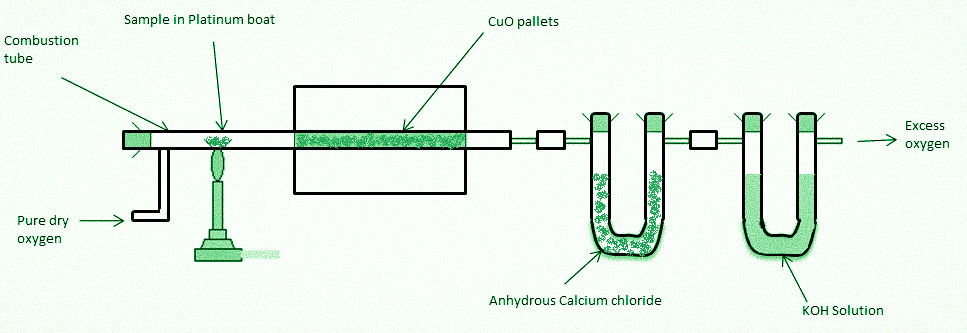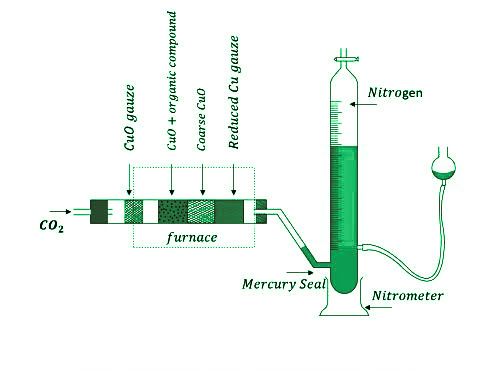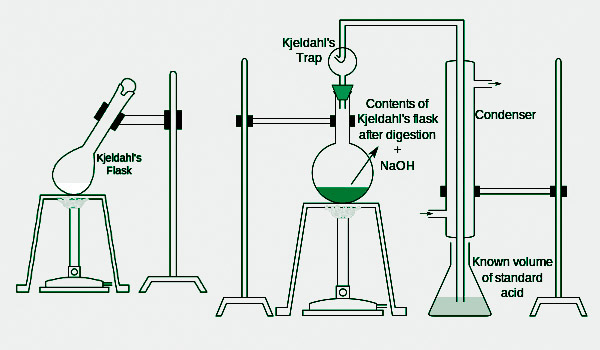GeeksforGeeks App
Open AppBrowser
Continue

## Related Articles

• CBSE Class 11 Chemistry Notes

# What is Quantitative Analysis?

Quantitative analysis is one of the important processes in chemistry. It is used to determine mass percent i.e. to determine the mass of every element present. It can also be defined as a method used to determine the number of chemicals in a sample. The mass per cent is important to find the molecular and emphatical formula.

The percentage of elements present in an organic compound can be determined by the following methods,

### Carbon and Hydrogen (Liebig’s method)

If we burn an organic compound in presence of excess oxygen and copper oxide, the carbon and hydrogen in the compound are oxidized to carbon dioxide and water respectively.

CxHy + (x + y)/4 O2 → x CO2 + (y/2) H2OIn the above process, the water and carbon dioxide formed on oxidation are absorbed by anhydrous calcium chloride and potassium hydroxide solutions present in both of the U-shaped tubes. This process gives the amounts of water and carbon dioxide from which the percentage of carbon and hydrogen are calculated.

Let us consider the mass of organic compound be mg, the mass of water and carbon dioxide produced be m1g and m2g respectively.

So, Percentage of carbon = (12 × m2 × 100) / (44 × m)

and Percentage of hydrogen = (2 × m1 × 100) / (18 × m)

### Nitrogen (Dumas Method and Kjeldahl’s method)

The percentage of nitrogen can be obtained through two methods namely

1. Dumas Method
2. Kjeldahl’s method

Dumas method: The organic compound containing nitrogen when heated with copper oxide in carbon dioxide, gives free nitrogen with carbon and water.

CxHyNz + (2x + y/2) CuO → xCO2 + y/2 H2O +z/2 N2 + (2x + y/2)CuIf any oxides of nitrogen are formed, they are reduced to nitrogen by passing the gaseous mixture over a heated copper gauze and is collected at the upper part of the tube. All such gases (Oxides of nitrogen) produced is brought to a solution of KOH which absorbs them.

Similar to the previous method,

Let, mg = mass of the organic compound,

V1 mL = Volume of nitrogen collect,

T1K = Room temperature.

So, Volume of Nitrogen at STP (standard temperature and pressure) =  (p1V1 × 273) / (760 × T1)

where,

• p1 = pressure of nitrogen,
• V1 = Volume of nitrogen.

Here, pressure is different from the atmospheric pressure at the place where nitrogen was collected. So the value of p1 is obtained by relation,

p1 = Atmospheric pressure – Aqueous tension

V mL N2 at STP = (28 ×  V/ 22400) × g

So, Percentage of Nitrogen = (28 × V × 100) / (22400 × m)

Kjeldahl’s method: The compound containing nitrogen is heated with concentrated H2SO4 (Sulphuric acid) which results in conversion of nitrogen compound to ammonium sulphate. The resulting acid mixture after the heating is then again heated with sodium hydroxide and ammonia gas is liberated. This liberated ammonia gas is absorbed in an excess of standard solution of Sulphuric acid. The amount of ammonia produced is determined by estimating the amount of sulphuric acid consumed in the reaction and the amount of sulphuric acid consumed is determined by the amount of sulphuric acid left unreacted (by titration with standard alkali solution).

Compound + H2SO4 → (NH4)2SO4 → (in presence of 2NaOH)

2NH3 + H2SO4 → (NH4)2SO4Let,

• mg = mass of the organic compound
• V mL = Volume of H2SO4 of molarity M
• V1 mL = Volume of NaOH of Molarity M (for titration)

V1 mL of NaOH of molarity M = V1/2 mL of H2SO4 = V1 mL

Volume of H2SO4 of molarity M unused = (V – V1/2) mL

(V – V1/2) mL  of H2SO4 of molarity M = 2(V -V1/2) mL of NH3 solution of molarity M.

1000 mL of 1M NH3 solution contains 17g NH3 or 14 g of N

2(V-V1/2) mL of NH3 solution of molarity M = [(14 × M × 2(V – V1/2)/1000)] g N

So, Percentage of N = [(14 × M × (V – V1/2))/1000] × (100/m)

N = 1.4 × M × 2 (V – V1/2) / m

1. Not applicable to compounds containing nitrogen in nitro and azo groups,
2. Not applicable for nitrogen present in Pyridine form (Ring).

### Halogens

Carius Method: A certain mass of an organic compound is heated with fuming nitric acid in presence of  silver nitrate contained in hard glass tube known as Carius tube and carbon and hydrogen present in compound are oxidized in carbon dioxide and water. The halogen present mix with the corresponding silver to form its halide (AgX) which is then filtered, dried and measured.

Let,

• mg = mass of the organic compound
• m1g = mass of AgX  (silver halide) formed

Here, 1 mol of AgX formed contains 1 mol of X.

Mass of halogen in m1g of AgX = (atomic mass of X × m1g) / molecular mass of AgX

Percentage of halogen = (atomic mass of X × m1 × 100) / molecular mass of AgX × m

### Sulphur

We again use the Carius tube in which we take a certain mass of an organic compound and heat it with sodium peroxide of fuming nitric acid. Sulphur present in the compound is oxidized to sulphuric acid. Then we need to add excess of barium chloride solution in water to precipitate the product as barium sulphate. The precipitate is filtered, dried, cleaned and measured.

Let,

• mg = mass of the organic compound,
• m1g = mass of barium sulphate formed

1 mol of BaSO4 = 233 g of BaSO4 = 32 g Sulphur

m1 g BaSO4 contains 32 × m1 g / 233 Sulphur

Percentage of sulphur = 32 × m1 × 100 / 233 × m

### Phosphorus

A certain mass of an organic compound is heated with fuming nitric acid where phosphorous present in the compound is oxidized into phosphoric acid. By adding ammonia and ammonia molybdate, it is precipitated as ammonium phosphomolybdate (NH4)3PO4.12MoO3 or alternatively, phosphoric acid may be precipitated as MgNH4PO4 by adding magnesia mixture which on ignition yields Mg2P2O7

Let,

• mg = mass of the organic compound,
• m1g = mass of ammonium phosphate molybdate

Molar mass of (NH4)3PO4.12MoO3 = 1877g

Percentage of phosphorus = (31×m1×100/1877×m) %

If instead of phosphorus, Mg2P2O7 was taken then,

Percentage of phosphorus = (62×m1×100/222×m) %

where,

• 222 u =  molar mass of Mg2P2O7
• m = mass of organic compound taken
• m1 = the mass of Mg2P2O7
• 62 = mass of two phosphorus atoms present in the compound Mg2P2O7.

### Oxygen

Every organic compound contains some percentage of oxygen in it. The percentage of oxygen can be calculated by the difference or total percentage of elements including Oxygen (100%) and the sum of all other elements present in the compound.

Alternatively, Oxygen can be emitted by chemical reaction as follows,

A certain mass of the organic compound is decomposed by heating in a stream of nitrogen gas. The mixture of gaseous products containing oxygen is passed over red hot coke when all the oxygen results in carbon monoxide. This mixture is passed through warm iodine pentoxide (I2O5) when carbon monoxide is oxidised to carbon dioxide which results in the production of iodine.

Organic compound → (In presence of heat) O2 + Other gases

2C + O2 → 2CO2

I2O5 + 5CO → I2 + 5CO2

Here we understand that each mole of oxygen released will give 2 moles of Carbon dioxide.

Thus if 32 g oxygen is released then 88 g carbon dioxide is obtained.

Let,

• mg = mass of organic compound
• m1g = mass of carbon dioxide released

m1g carbon dioxide is obtained from (30×m/88×m)%

The percentage of oxygen can be derived from the amount of Iodine produced.

### Sample Problems

Problem 1: On Complete combustion, 0.456 g of an organic compound gave 0.254 of carbon dioxide and 0.1658 of water. Determine the percentage composition of carbon and hydrogen in the compound.

Solution:

Percentage of carbon = (12×0.254×100)/(44×0.456)

∴ Percentage of  carbon = 15.1914 %

and Percentage of Hydrogen = (2×0.1658×100)/(18×0.456)

∴Percentage of Hydrogen = 4.03 %

Problem 2: In the dumas method for estimation of nitrogen, 0.3 g of an organic compound gave 50 mL of nitrogen collected at 300 K temperature and 715 mm pressure. Calculate the percentage composition of nitrogen in the compound. (Tension of the aqueous medium is = 15 mm)

Solution:

Given :

• Volume of nitrogen = 50mL
• Temperature = 300 K
• Pressure = 715 mm
• Actual pressure = 715 – 15 = 700 mm

Volume of Nitrogen at STP = (273×700×50)/(300×760) = 41.9 mL,

22,400 mL of N2 at STP weighs 28 g

41.9 mL of Nitrogen weighs = (28×41.9)/22400 g

∴Percentage of Nitrogen = 17.46 %.

Problem 3: In the Carius method of estimation of halogen, 0.20 g of an organic compound gave 0.16 g of AgBr (silver bromide). Find out the percentage of bromine in the compound.

Solution:

Molar mass of AgBr = 108 + 80 =188 g mol-1

188 g AgBr contains 80 g bromine,

0.16 g AgBr contains (80×0.16)/ 188 g bromine,

Percentage of bromine = (80×0.16×100)/( 188×0.20)

∴ Percentage of bromine = 34.04 %

Problem 5: In sulphur estimation, 0.210 g of an organic compound gave 0.5101 g of barium sulphate. What is the percentage of sulphur in the compound?

Solution:

Molecular mass of BaSO4 = 137+32+64 = 233 g,

233 g BaSO4 contains 32 g sulphur,

0.5010 g BaSO4 contains (32×0.5010)/233 g sulphur,

Percentage of sulphur = (32×0.5010×100)/(233×0.210)

∴Percentage of sulphur = 32.76 %

Problem 5: During the estimation of nitrogen present in an organic compound by Kjeldahl’s method, the ammonia evolved from 0.8 g of the compound in Kjeldahl’s estimation of nitrogen, neutralized 20 mL of 1M H2SO4. Find out the percentage of nitrogen in the compound.

Solution:

1M of 20 mL H2SO4 = 1M of 40 mL,

NH3 1000 mL of 1M ammonia contains 14 g of nitrogen,

40 mL of 1M ammonia contains (14×40)/1000,

Percentage of nitrogen = (14×40×100)/(1000×0.8),

∴Percentage of Nitrogen = 70.0%

### Conceptual Questions

Question 1: Explain what happens in Liebig’s method.

The water and carbon dioxide formed on oxidation are absorbed by anhydrous calcium chloride and potassium hydroxide solutions present in both of the U-shaped tubes. This process gives the amounts of water and carbon dioxide from which the percentage of carbon and hydrogen are calculated.

Question 2: State and define the Quantitative analysis methods of Nitrogen.